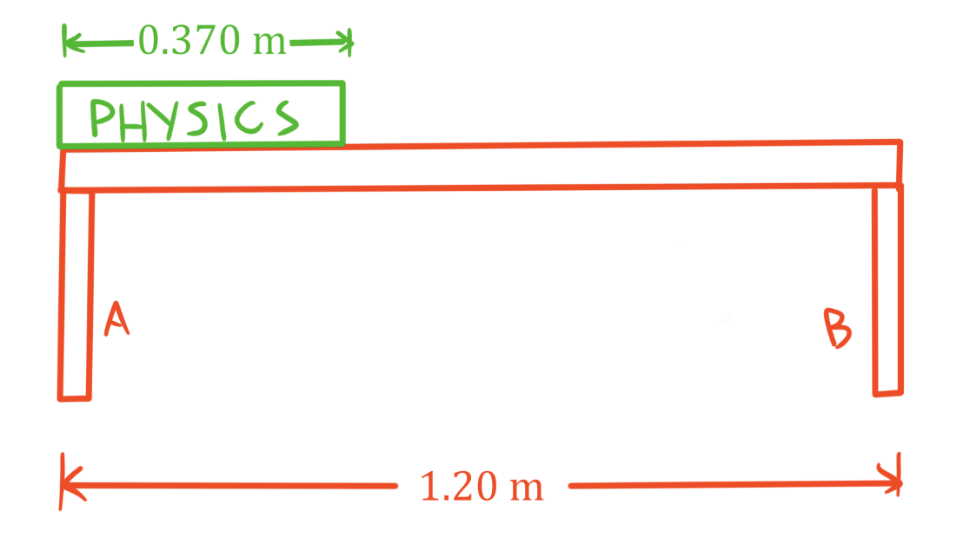Rotational equilibrium

Rotational equilibrium

Lessons

In this lesson, we will learn:

• Meaning of rotational equilibrium
• Solving problems involving rotational equilibrium

Notes:

• An object can undergo translational motion (motion that changes its position) and rotational motion (motion that changes its angle).
• An object is in rotational equilibrium if the velocity of its rotation is constant. An object that is not rotating or an object that is rotating in one direction a constant rate would be considered in rotational equilibrium. To be in rotational equilibrium, the net torque acting on the object must be zero.
• Torque is twisting force: it is a vector quantity that describes how much a force acting on an object tends to make that object rotate.
• The torque produced by a force depends on how far the force is applied from the point of rotation of the object, which is called the pivot. A force that is applied close to the pivot produces less torque than an equal magnitude force applied further away from the pivot. The distance from the pivot to where the force is applied is called the lever arm.
• Only a force that is perpendicular to distance from the pivot can generate a torque. If a force at a non-perpendicular angle creates a torque, only the perpendicular component of the force contributes to the torque.
• It is important to consider the force of gravity acting on an object that is in rotational equilibrium, since this force can generate torque.
• Gravity acts on the entire object (that is, gravity pulls on an object at every point inside that object). When solving problems involving gravity we simplify this by imagining the entire force of gravity acts on a single point in the object called the center of gravity.
• For an object affected by uniform gravity (such as an object influenced by Earth's gravity), the center of gravity is located at the same point as the center of mass. The center of mass can be thought average position of mass in the object.
• For an object with uniform density and with a simple shape (like a rod, plank, cube, sphere, disc, etc.) the center of mass is located at the geometric center of the object.
• Center of mass is not necessarily in the geometric center of objects with non-uniform density (denser at some parts than others) or with irregular shapes.
• Finding the center of mass/center of gravity allows us to find the distance away from the pivot that gravity acts at and allows us to calculate the torque caused by gravity.
Torque

$\tau = F_{\perp}d$

$\tau$: torque, in newton meters (N·m)

$F_{\perp}:$ component of force perpendicular to $d$, in newtons (N)

$d:$ distance from point of rotation, in meters (m)

Conditions for Rotational Equilibrium

$\Sigma \tau = 0$ N·m

or simpler equation:

total $CW$ $\tau$ = total $CCW$ $\tau$

$\Sigma \tau :$ sum of all torques, in newton meters (N·m)

total $CW$ $\tau$: magnitude of all torques in the clockwise direction, in newton meters (N·m)

total $CCW$ $\tau$: magnitude of all torques in the counterclockwise direction, in newton meters (N·m)

• 1.
Rotational equilibrium of masses balanced on a single pivot point

A uniform 1.10 kg rod is balanced on a pivot and two masses of 2.50 kg and 1.25 kg are hung as shown. At what distance away from the pivot should the 1.25 kg mass be hung so that the two masses balance?• 2.
Rotational equilibrium with two pivot points

A 1.75 kg uniform book rests on a 1.10 kg uniform horizontal shelf as shown. What is the force exerted on the shelf by each support A and B?